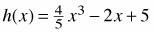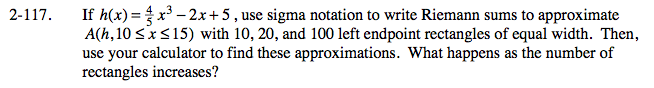### Home > CALC > Chapter 2 > Lesson 2.3.2 > Problem2-117

2-117.

If, use sigma notation to write Riemann sums to approximate A(h, 10 ≤ x ≤ 15) with 10, 20, and 100 left endpoint rectangles of equal width. Then, use your calculator to find these approximations. What happens as the number of rectangles increases? Homework Help ✎10 left-endpoint rectangles:

20 left-endpoint rectangles:

100 left-endpoint rectangles: